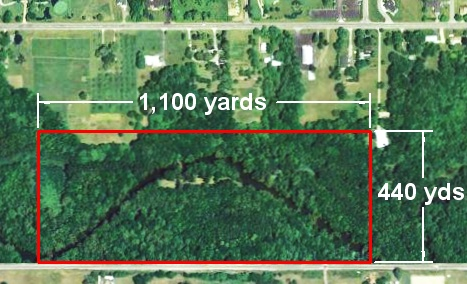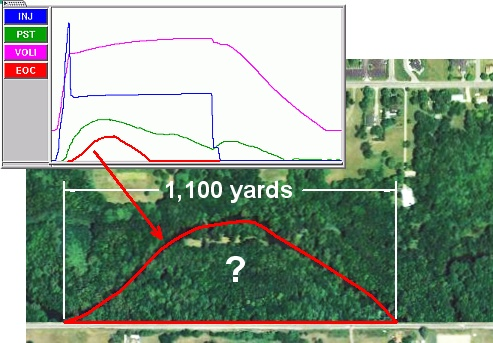Select Page

We regularly struggle to explain what an “integral” is and where it comes from. Integrals are numbers calculated by the eDART that are particularly useful for detecting changes in part weight or dimensions.

Generally we say an integral is the “area under the curve,” thinking that should settle it. But it still leaves some people confused. Many people can visualize area for a rectangle as length x width. But what if you are thrown a curve?

Here is a little exercise that may help with this area concept. Let us start with a section of land bounded by a road and some survey lines that looks like the outline here.It is easy to calculate the area of this land: 440 yards x 1,100 yards = 484,000 square yards (yards x yards = “square yards”). There are 4,840 square yards in an acre so this is exactly 100 acres of land (484,000 ÷ 4,840).

But suppose you only own from the road to the river. This bend in the river looks suspiciously like a cavity pressure curve.You really don’t want to pay property taxes on the hundred acre rectangle when you only own to the river. The actual area of this property is the “area under the curve”; that is, inside that boundary of the road and the river. The eDART’s™ lower boundary (the road) is zero pressure (bottom of the cycle graph) and the upper is the pressure curve.

So what is the area under that curve or inside that property boundary? It turns out to be about 45 acres.

Next tip ( # 149 ): How do we compute the area inside that curve?